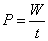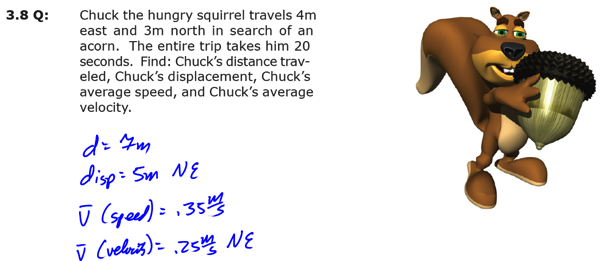## Vectors and Scalars## LAB: Speeding on Cooper Road## Defining PowerPower is a term used quite regularly in all aspects of life. We talk about how powerful the new boat motor is, the power of positive thinking, and even the power company’s latest bill. All of these uses of the term power relate to how much work can be done in some amount of time.

In physics, work is the process of moving an object by applying a force. The rate at which the force does work is known as power (P). The units of power are the units of work divided by time, or Joules per second, known as a watt (W).Since power is the rate at which work is done, it is possible to have the same amount of work done but with different supplied power, if the time is different.

Question: Jennifer pushes a sofa 3 meters across the floor by applying a force of 200N. If it takes her 6 seconds to push the sofa, what amount of power did she supply?

Answer:Question: Kevin pushes the same sofa 3 meters across the floor by applying a force of 200N. Kevin, however, takes 12 seconds to push the sofa. What amount of power did Kevin supply?

Answer:As you can see, although Jennifer and Kevin both did 600J of work in pushing the softa, Jennifer supplied twice the power of Kevin because she did the same work in half the time!

## Calculating Power

There’s more to the story, however. Since power is defined as work over time, we can write the equation for power as:And because work is equal to force (in the direction of the displacement) multiplied by displacement, we can replace work in the equation with F*d:Looking carefully at this equation, you can observe a displacement divided by time. Since displacement divided by time is the definition of average velocity, we can replace d/t with v in the equation to obtain:So, not only is power equal to work done divided by the time required, it’s also equal to the force applied (in the direction of the displacement) multiplied by the velocity of the object.

Question: Motor A lifts a 5000N steel crossbar upward at a constant 2 m/s. Motor B lifts a 4000N steel support upward at a constant 3 m/s. Which motor is supplying more power?

Answer: Motor B supplies more power, as shown below:## Distance, Displacement, Speed, Velocity